# On overlapping error bars in charts

Andrew Gelman posted an example graph the other day in a blog post which showed trends over time in measures of smiling in high school yearbook photos.Surprisingly, Andrew did not make a comment on the error bars in the graph. Error bars with cross hairs are often distracting in the plot. In the example graph it is quite bad, in that they perfectly overlap, so the ends are very difficult to disentangle. Here I will suggest some alternatives.

I simulated data that approximately captures the same overall trends, and replicated the initial chart in SPSS.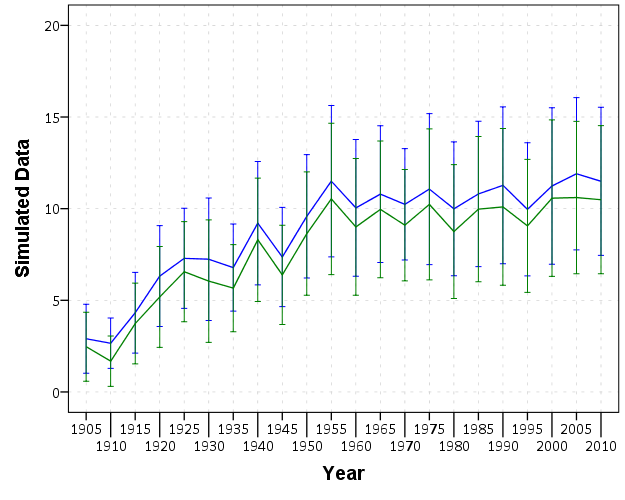First, a simple solution with only two groups is to use semi-transparent areas instead of the error bars.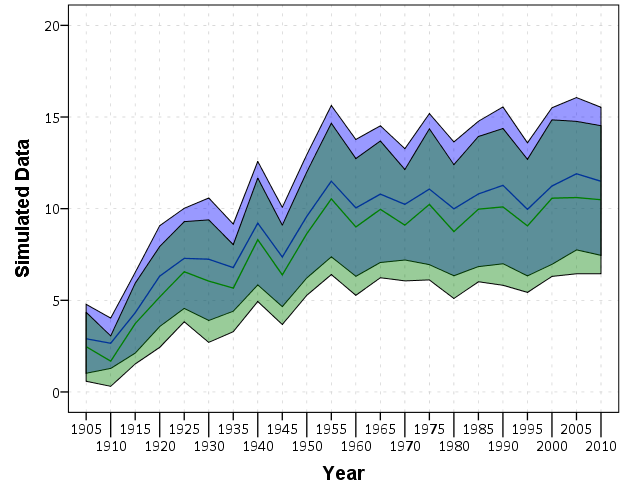This makes it quite easy to see the overlap and non-overlap of the two groups. This will even print out nice in black-white. In the end, this chart is over-complicated by separating out genders. Since each follow the same trend, with females just having a constant level shift over the entire study period, there is not much point in showing each in a graph. A simpler solution would just pool them together (presumably the error bars would be smaller by pooling as well). The advice here still applies though, and the areas are easier to viz. than the discontinuous error bars.

For more complicated plots with more groups, I would suggest doing small multiples.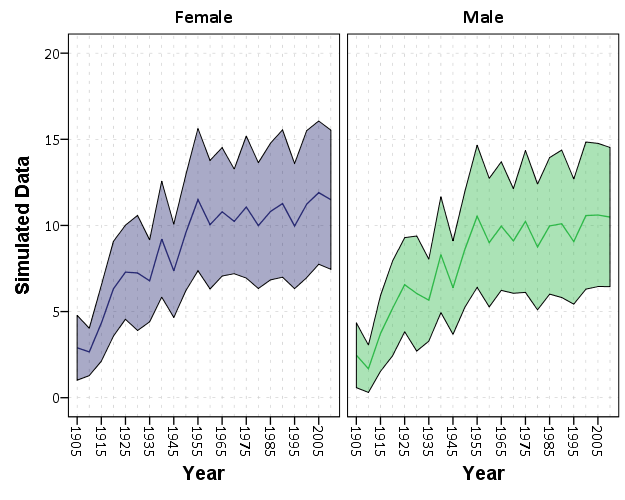While it is harder now to see the exact overlap between groups, we can at least visually assess the trends within each group quite well. In the original it is quite a bit of work to figure out the differences between groups and keep the within group comparisons straight. Since the trends are so simple it is not impossible, but with more variable charts it would be quite a bit of work.

For instances in which a trend line is not appropriate, you can dodge the individual error bars on the x-axis so that they do not perfectly overlap. This is the same principle as in clustered bar charts, just with points and error bars instead of bars.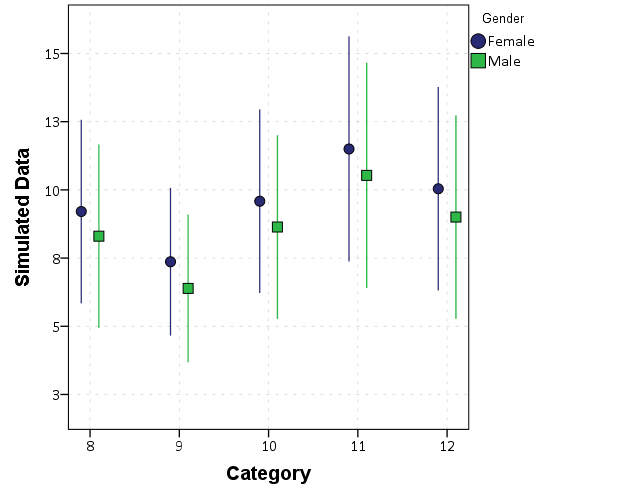Here I like using just the straight lines (a tip taken from Andrew Gelman). The serif part of the I beam like error bars I find distracting, and make it necessary to separate the lines further. Using just the lines you can pack many more into a small space, like caterpillar plots of many random effects.

Here is a copy of the SPSS syntax used to generate these graphs.

# Using and Making Cumulative Probability Charts

Stephen Few had a recent post critiquing an evaluation of a particular data visualization. Long story short, the experiment asked questions like “What is the probability that X is above 5?”, and showed the accuracy based on mean+error bar charts, histogram like visualizations, and animated vizualations showing random draws.

It is always the case in data viz. that some charts are easier to answer particular questions. This is one question, what is the probability a value is above X, in which traditional histograms or error bar charts are not well suited for. But there is an alternative I don’t see used very often, the cumulative probability chart, that is well suited to answer that question.

It is a totally reasonable question to ask as well. For one example use when I was a crime analyst, I used this chart to show the time in-between shootings. Many shootings are retaliatory so I was interested in saying if a shooting happened on Sunday, how long should be PD be on guard for after an initial shooting. Do most retaliatory shootings happen within hours, days, or weeks of a prior shooting? This is a hard question to answer with histograms, but is easier to answer with cumulative probability plots.

Here is that example chart for time-in-between shootings: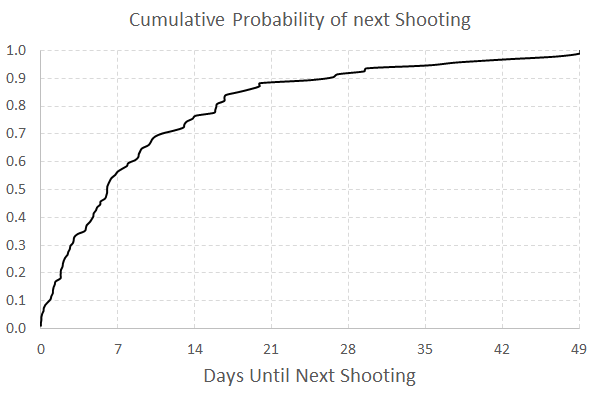Although this chart is not regularly used, it is really easy to explain how to interpret. For example, at time equals 7 days (on the X axis), the probability that a shooting would have occurred is under 60%. In my opinion, it is easier to explain this chart than a histogram to a lay audience.

To produce the chart it is often not a canned option in software, but it takes very simple set of steps to produce the right ingrediants – and then you can use a typical line chart. So those steps generically are:

• sort the data
• rank the data (1 for the lowest value, 2 for the second lowest value, etc.)
• calculate rank/(total sample size) – call this `Prop`
• plot the data on the X axis, and `Prop` on the Y axis

Which can be easily done in any software, but here you can download an excel spreadsheet here used to make the above chart.

A variant of this chart often used in crime analysis is the proportion of places on the X axis and the cumulative proportion of crime on the Y axis. E.g. Pareto’s 80/20 rule – or 50/1 rule – or whatever. The chart makes it easy to pick whatever cut-offs you want. If you have your spatial units of analysis in one column, and the total number of crimes in a second column, the procedure to produce this chart is:

• sort the data descending by number of crimes
• rank the data
• calculate rank/(total sample size) – this equals the proportion of all spatial units – call this `PropUnits`
• calculate the cumulative number of crimes – call this Cum_Crime
• calculate Cum_Crime/(Total Crime) – this equals the proportion of all crimes – call this `PerCumCrime`
• plot `PerCumCrime` on the Y axis and `PropUnits` on the X axis.

See the third sheet of the excel file for a hypothetical example. This pattern basically happens in all aspects of criminal justice. That is, the majority of the bad stuff is happening among a small number of people/places. See this example from William Spelman showing places, victims, and offenders.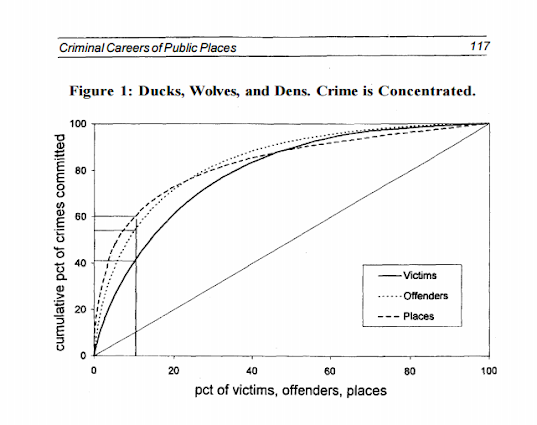We can see there that 10% of the victims account for 40% of all victimizations etc.

# Maps in inline GPL statements (SPSS)

Here I will go through an example of using inline GPL statements to import map backgrounds in SPSS charts. Here you can download the data and code to follow along with this post. This is different than using maps via VIZTEMPLATE, as I will show.

Note you can also use the graphboard template chooser to make some default maps, but I’ve never really learned how to make them on my own. For example, say I want to map that sets both the color and the transparency of areas based on different attributes. This is not possible with the current selection of map templates that comes with SPSS (V22).

But I figured out some undocumented ways to import maps into inline GPL code, and you can get pretty far with just the possibilities available within the grammar of graphics.

The data I will be using is a regular grid of values across DC. What I calculated was the hour of the day with the most Robberies over along time period (2011 through 2015 data) using a weighted average approach synonymous with geographically weighted regression. Don’t take this too seriously though, as there appears to be some errors in the time fields for the historical DC crime data.

So below I first define a handle to where my data is stored, recode the hour field into a smaller set of bins, and then make a scatterplot.

``````FILE HANDLE data /NAME = "C:\Users\andrew.wheeler\Dropbox\Documents\BLOG\Inline_Maps_GGRAPH".

GET FILE = "data\MaxRobHour.sav".
DATASET NAME MaxRob.

*Basic Scatterplot.
FREQ HourEv.
RECODE HourEv (0 THRU 3 = 1)(11 THRU 19 = 2)(ELSE = COPY) INTO HourBin.
VALUE LABELS HourBin
1 '0 to 3'
2 '11 to 19'.

DATASET ACTIVATE MaxRob.
* Chart Builder.
GGRAPH
/GRAPHDATASET NAME="graphdataset" VARIABLES=XMetFish[LEVEL=SCALE] YMetFish[LEVEL=SCALE] HourBin
MISSING=LISTWISE REPORTMISSING=NO
/GRAPHSPEC SOURCE=INLINE.
BEGIN GPL
SOURCE: s=userSource(id("graphdataset"))
DATA: XMetFish=col(source(s), name("XMetFish"))
DATA: YMetFish=col(source(s), name("YMetFish"))
DATA: HourBin=col(source(s), name("HourBin"), unit.category())
GUIDE: axis(dim(1), label("XMetFish"))
GUIDE: axis(dim(2), label("YMetFish"))
GUIDE: legend(aesthetic(aesthetic.color.exterior), label("HourBin"))
ELEMENT: point(position(XMetFish*YMetFish), color.exterior(HourBin))
END GPL.``````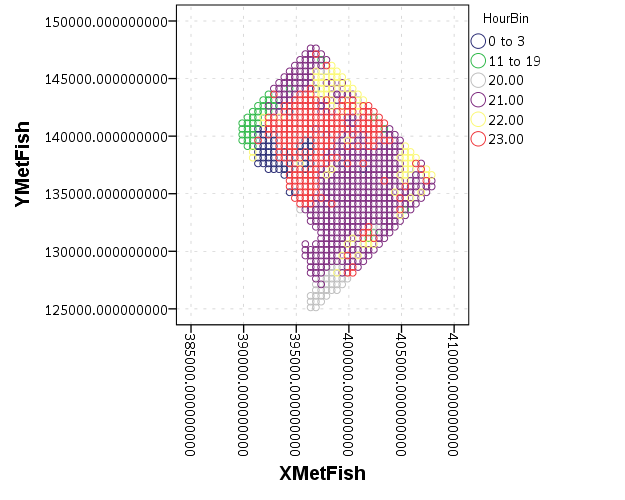We can do quite a bit to make this map look nicer. Here I change:

• make the aspect ratio 1 to 1, and set the map limits
• get rid of the X and Y axis (the particular projected coordinates make no difference)
• make a nice set of colors based on a ColorBrewer palatte and map the color to the interior of the point

And below that is the map it produces.

``````*Making chart nice, same aspect ratio, colors, drop x & y.
FORMATS HourBin (F2.0).
GGRAPH
/GRAPHDATASET NAME="graphdataset" VARIABLES=XMetFish[LEVEL=SCALE] YMetFish[LEVEL=SCALE] HourBin
MISSING=LISTWISE REPORTMISSING=NO
/GRAPHSPEC SOURCE=INLINE.
BEGIN GPL
SOURCE: s=userSource(id("graphdataset"))
DATA: XMetFish=col(source(s), name("XMetFish"))
DATA: YMetFish=col(source(s), name("YMetFish"))
DATA: HourBin=col(source(s), name("HourBin"), unit.category())
COORD: rect(dim(1,2), sameRatio())
GUIDE: axis(dim(1), null())
GUIDE: axis(dim(2), null())
GUIDE: legend(aesthetic(aesthetic.color.exterior), label("HourBin"))
SCALE: linear(dim(1), min(389800), max(408000))
SCALE: linear(dim(2), min(125000), max(147800))
SCALE: cat(aesthetic(aesthetic.color.interior), map(("1",color."810f7c"),("2",color."edf8fb"),("20",color."bfd3e6"),("21",color."9ebcda"),
("22",color."8c96c6"),("23",color."8856a7")))
ELEMENT: point(position(XMetFish*YMetFish), color.interior(HourBin))
END GPL.``````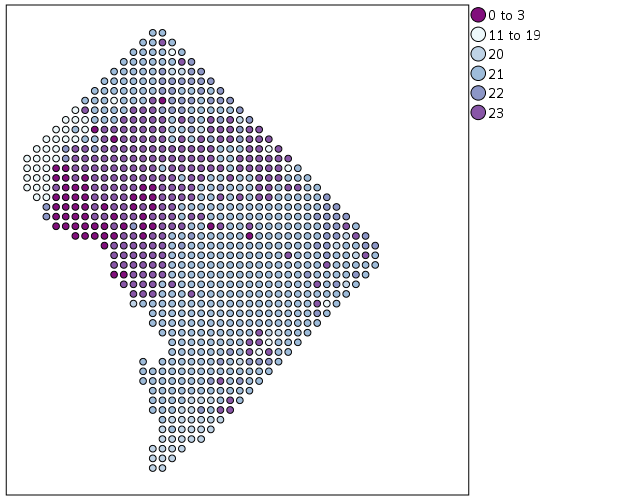So that is not too shabby a map for just plain SPSS. Now it is a bit hard to vizualize the patterns though, because the surface has needless discontinuities because of the circles. We can use squares as the shape and just do some experimentation to figure out the size needed to fill up each grid cell. Also pro-tip when making choropleth maps, with many areas often light outlines look nicer than black ones.

``````*Alittle nicer, squares, no outline.
GGRAPH
/GRAPHDATASET NAME="graphdataset" VARIABLES=XMetFish[LEVEL=SCALE] YMetFish[LEVEL=SCALE] HourBin
MISSING=LISTWISE REPORTMISSING=NO
/GRAPHSPEC SOURCE=INLINE.
BEGIN GPL
SOURCE: s=userSource(id("graphdataset"))
DATA: XMetFish=col(source(s), name("XMetFish"))
DATA: YMetFish=col(source(s), name("YMetFish"))
DATA: HourBin=col(source(s), name("HourBin"), unit.category())
COORD: rect(dim(1,2), sameRatio())
GUIDE: axis(dim(1), null())
GUIDE: axis(dim(2), null())
GUIDE: legend(aesthetic(aesthetic.color.exterior), label("HourBin"))
SCALE: linear(dim(1), min(389800), max(408000))
SCALE: linear(dim(2), min(125000), max(147800))
SCALE: cat(aesthetic(aesthetic.color.interior), map(("1",color."810f7c"),("2",color."edf8fb"),("20",color."bfd3e6"),("21",color."9ebcda"),
("22",color."8c96c6"),("23",color."8856a7")))
ELEMENT: point(position(XMetFish*YMetFish), color.interior(HourBin), shape(shape.square), size(size."9.5"),
transparency.exterior(transparency."1"))
END GPL.``````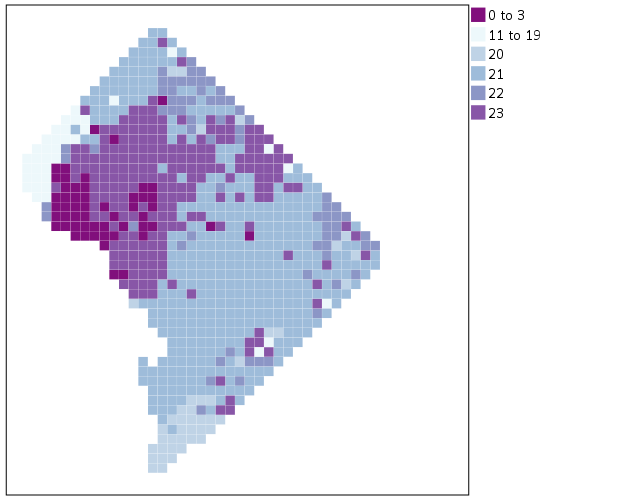Again looking pretty good for just a map in plain SPSS. With the larger squares it is easier to clump together areas with similar patterns for the peak robbery time. The city never sleeps in Georgetown it appears. A few of the polygons though are very hard to see on the edge of DC though, so we will add in the outline. See the `SOURCE: mapsrc`, `DATA: lon*lat`, and the `ELEMENT: polygon` lines for how this is done. The “DCOutline.smz” is the map template file created by SPSS.

``````*Now include the outline.
GGRAPH
/GRAPHDATASET NAME="graphdataset" VARIABLES=XMetFish[LEVEL=SCALE] YMetFish[LEVEL=SCALE] HourBin
MISSING=LISTWISE REPORTMISSING=NO
/GRAPHSPEC SOURCE=INLINE.
BEGIN GPL
SOURCE: s=userSource(id("graphdataset"))
DATA: XMetFish=col(source(s), name("XMetFish"))
DATA: YMetFish=col(source(s), name("YMetFish"))
DATA: HourBin=col(source(s), name("HourBin"), unit.category())
SOURCE: mapsrc = mapSource(file("C:\\Users\\andrew.wheeler\\Dropbox\\Documents\\BLOG\\Inline_Maps_GGRAPH\\DCOutline.smz"))
DATA: lon*lat = mapVariables(source(mapsrc))
COORD: rect(dim(1,2), sameRatio())
GUIDE: axis(dim(1), null())
GUIDE: axis(dim(2), null())
GUIDE: legend(aesthetic(aesthetic.color.exterior), label("HourBin"))
SCALE: linear(dim(1), min(389800), max(408000))
SCALE: linear(dim(2), min(125000), max(147800))
SCALE: cat(aesthetic(aesthetic.color.interior), map(("1",color."810f7c"),("2",color."edf8fb"),("20",color."bfd3e6"),("21",color."9ebcda"),
("22",color."8c96c6"),("23",color."8856a7")))
ELEMENT: point(position(XMetFish*YMetFish), color.interior(HourBin), shape(shape.square), size(size."9.5"),
transparency.exterior(transparency."1"))
ELEMENT: polygon(position(lon*lat))
END GPL.``````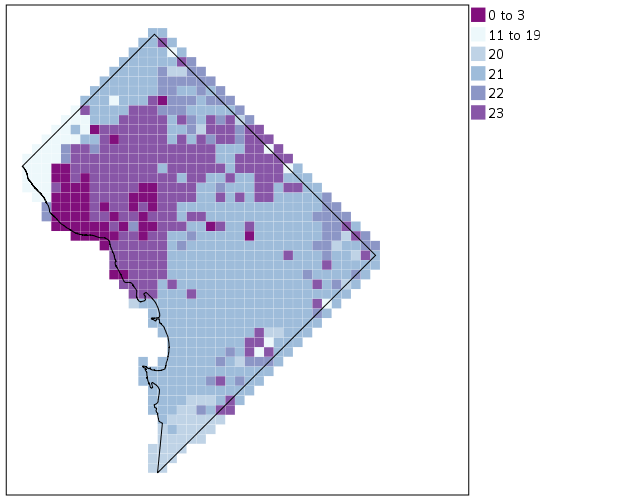Now we have a bit more of a reference. The really late at night area appears to be north of Georgetown. The reason I figured this was even possible is that although `mapSource` is not documented in the GPL reference guide, there is an example using it with the `project` function (see page 194).

Now, if I were only making one map this isn’t really much of a help – I would just export the data values, make it in ArcGIS and be done with it. But, one of the things hard to do in GIS is make small multiple maps. That is something we can do fairly easily in stat. software though. For an example, here I make a random map to compare with the observed patterns. The grammar automatically recognizes `lon*lat*Type` and replicates the background outline across each panel. Also I change the size of the overall plot using `PAGE` statements. I just typically experiment until it looks nice.

``````*Can use the outline to do small multiples.
COMPUTE HourRand = TRUNC(RV.UNIFORM(0,24)).
RECODE HourRand (0 THRU 3 = 1)(4 THRU 19 = 2)(ELSE = COPY).
VARSTOCASES
/MAKE Hour FROM HourBin HourRand
/INDEX Type.
VALUE LABELS Type 1 'Observed' 2 'Random'.

*Small multiple.
GGRAPH
/GRAPHDATASET NAME="graphdataset" VARIABLES=XMetFish YMetFish Hour Type
MISSING=LISTWISE REPORTMISSING=NO
/GRAPHSPEC SOURCE=INLINE.
BEGIN GPL
PAGE: begin(scale(1000px,500px))
SOURCE: s=userSource(id("graphdataset"))
DATA: XMetFish=col(source(s), name("XMetFish"))
DATA: YMetFish=col(source(s), name("YMetFish"))
DATA: Hour=col(source(s), name("Hour"), unit.category())
DATA: Type=col(source(s), name("Type"), unit.category())
SOURCE: mapsrc = mapSource(file("C:\\Users\\andrew.wheeler\\Dropbox\\Documents\\BLOG\\Inline_Maps_GGRAPH\\DCOutline.smz"))
DATA: lon*lat = mapVariables(source(mapsrc))
COORD: rect(dim(1,2), sameRatio(), wrap())
GUIDE: axis(dim(1), null())
GUIDE: axis(dim(2), null())
GUIDE: axis(dim(3), opposite())
GUIDE: legend(aesthetic(aesthetic.color.exterior), label("HourBin"))
SCALE: linear(dim(1), min(389800), max(408000))
SCALE: linear(dim(2), min(125000), max(147800))
SCALE: cat(aesthetic(aesthetic.color.interior), map(("1",color."810f7c"),("2",color."edf8fb"),("20",color."bfd3e6"),("21",color."9ebcda"),
("22",color."8c96c6"),("23",color."8856a7")))
ELEMENT: point(position(XMetFish*YMetFish*Type), color.interior(Hour), shape(shape.square), size(size."8"),
transparency.exterior(transparency."1"))
ELEMENT: polygon(position(lon*lat*Type))
PAGE: end()
END GPL.``````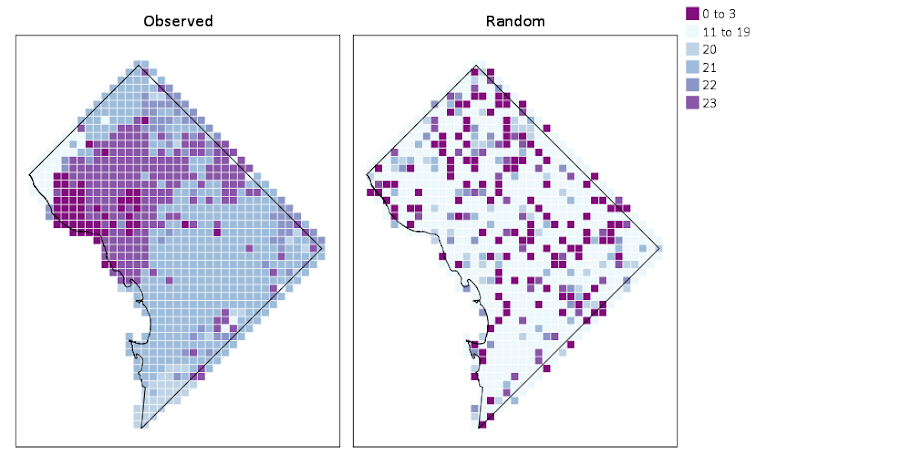We can see that this extreme amount of clustering is clearly not random.

This example works out quite nice because the micro level areas are a regular grid, so I can simulate a choropleth map look by just using square point markers. Unfortunately, I was not able to figure out how to map areas to merge a map file and an id like you can in VIZTEMPLATE. You can see some of my attempts in the attached code. You can however have multiple `mapSource` statements, so you could import say a street network, rivers and parks and map a nice background map right in SPSS. Hopefully IBM updates the documentation so I can figure out how to make a choropleth map in inline GPL statements.

# Keeping it simple: Viz. mass shooting definitions

My wife asked me the other day about some mass shooting statistics, in particular some claims of an average of one a day in the US. Without knowing the source, I told her outright it is probably because that person widened the net to events beyond what most people stereotypically consider a mass shooting.

Now, I have no personal opinion on how it should be defined, and being a researcher in criminal justice I appreciate people digging into the details. I was prompted to write this post by an interactive application showing how the numbers change by Kevin Schaul of the Washington Post (referred via Flowing Data). I was pretty frustrated by Kevin’s example interactive application though – there are much simpler ways than making me change the definition and seeing what individual events pop up. Here is an example screen shot of inputting a definition and then how Kevin’s data pop out.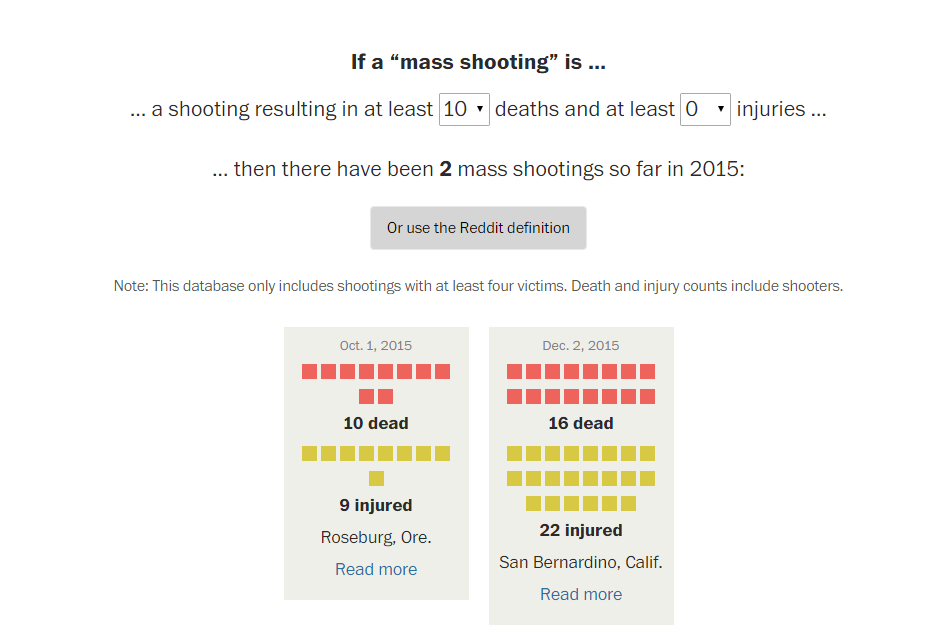So, downloading the same Reddit data for 2015 so far (as of 12/7/15) I created what I consider to be simple summaries. Caveat – these crowdsourced datasets are likely to have substantial missing data, especially towards the events with fewer injured. First I made a frequency histogram of the total number of dead per incident.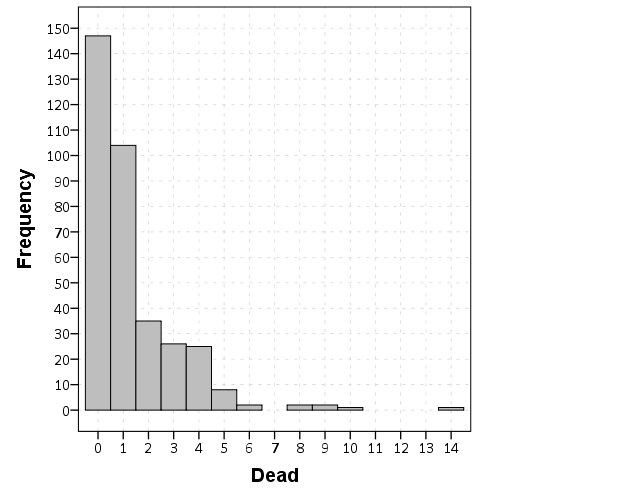So you can see that if you only want to include dead in your personal definition, the one per day statistic is a dramatic over-representation. If you want to draw the line at 5 or more you will have around 9 more events than you would if you made the line at 6 or more. If you make the line at 10 or more there are only two incidents, but there are another 4 if you include incidents with 8 or 9 dead.

Another simple overview is a table. Here are tables of dead, injured, and the combined counts per each incident, sorted in descending value of the count. So the way to read this is that there there 147 seperate incidents in the reddit database that had 0 deaths, and 104 that had only one death, etc. The tables also have percents and cumulative percentage, so you can see how where you define the cut-point changes how much of the data you chop-off. Cumulative counts would be just as useful.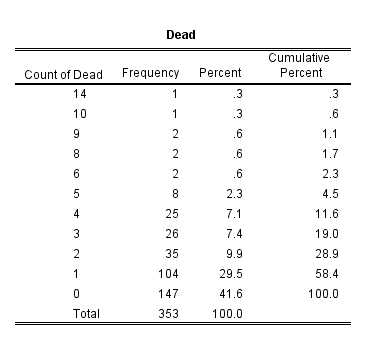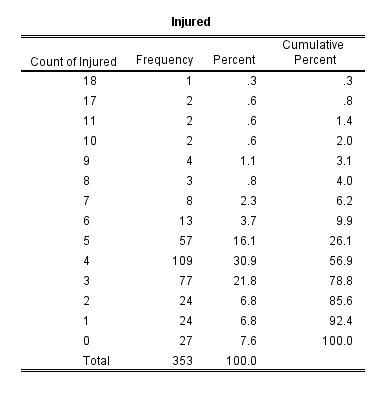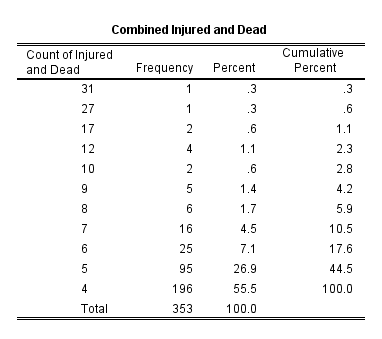I have no personal problem using injured as well in a mass shooting definition. Basically the difference between being shot and being killed is seemingly due to random happenstance, so a shooting with 10 injured and no one killed can easily be argued to be a mass shooting in my opinion. Kevin’s interactive makes you choose an and condition though between injured and killed, whereas one could place the cut point at an or condition or simply the combined total. Here is a cross tabulation of the frequencies of injured by dead.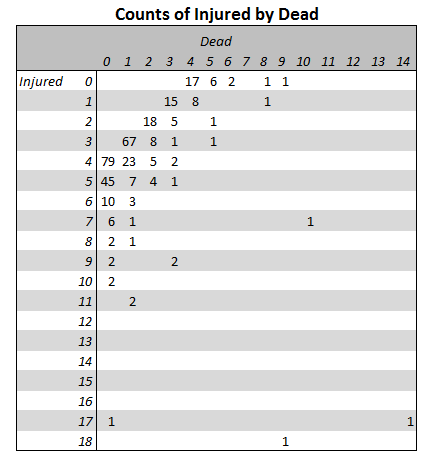You can clearly see the reddit definition is the combined total of injured or dead is 4 via the line on the upper left of the table. Kevin’s and condition forces you to make a cut-point along each axis, basically choosing a rectangle in the lower right of the above crosstab table. If you want a combined total though, it will be along a diagonal somewhere in the table.

I appreciate these interactive visualizations allow a viewer to dig deeper into specific events in the data, but that does not mean some simple summaries could not also accompany the piece.

# Poster presentations should have a minimum font size of 25 points

A fairly generic problem I’ve been trying to do some research on is how large should fonts be for posters and PowerPoint presentations. The motivation is my diminishing eyesight over the years, and in particular default labels for statistical graphics are almost always too small in my opinion. Projected presentations just exacerbate the problem.

First, to tackle the project we need to find research about the the sizes that individuals can comfortably read letters. You don’t measure size of letters in absolute distance terms though, you measure it in the subtended angle that an object commands in your vision. That is, it is both a function of the height of the letters as well as the distance you are away from the object. I.e. in the below diagram angle A is larger than angle B.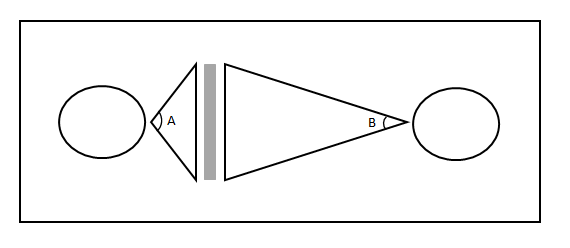The best guide for the size of this angle I have found for letters is an article by Sidney Smith, Letter Size and Legibility. Smith (1979) had a set of students make various labels and then have people stand too far away to be able to read them. Then the participants walked towards the labels until they could read them. Here is the histogram of those subtended angles (in radians) Smith produced: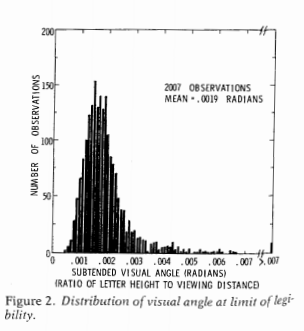From this Smith gives the recommendation as 0.007 radians as a good bet for pretty much everyone to be able to read the text. My research into other recommendations (eye tests, highway symbols) tends to be smaller, and between mine and Smith’s other sources tends to produce a range of 0.003 to 0.010 radians. Personal experimentation for me is that 0.007 is a good size, although up to 0.010 is not uncomfortably large. Most everyone with corrective vision can clearly see under 0.007, but we shouldn’t be making our readers strain to read the text.

For comparison, I sit approximately 22 inches away from my computer screen. A subtended angle of 0.007 produces a font size of just over 11 points at that distance. At my usual sitting distance I can read fonts down to 7 points, but I would prefer not to under usual circumstances.

This advice can readily translate to font sizes in poster presentations, since there is a limited range in which people will attempt to read them. Block’s (1996) suggestion that most people are around 4 feet away when they read a poster seems pretty reasonable to me, and so this produces a letter height of 0.34 inches needed to correspond to a 0.007 subtended angle. One point of font is 1/72 inches in letter height, so this converts to a 25 point font as the minimum to which most individuals can comfortably read the words in a poster. (R Functions at the end of the post for conversions, although it is based on relatively simple geometry.)

This advice is larger than Block’s (which is 20 point), but fits in line with Colin Purrington’s templates, which use 28 point for the smallest font. Again note that this is the minimum font for the poster, things like titles and author names should clearly be larger than the minimum to create a hierarchy. Again a frequent problem are axis labels for statistical graphics.

It will take more work to extend this advice to projected presentations, since there is more variability in projected sizes as well as rooms. So if you see a weirdo with a measuring tape at the upcoming ASC conference, don’t be alarmed, I’m just collecting some data!

Here are some R functions, the first takes a height and distance and return the subtended angle (in radians). The second takes the distance and radians to produce a height.

``````visual_angleR <- function(H,D){
x <- 2*atan(H/(2*D))
return(x)
}

return(x)
}``````

Since a point of font is 1/72 of an inch, the code to calculate the recommended font size is `visual_height(D=48,Rad=0.007)*72` and I take the ceiling of this value for the 25 point recommendation.

# Custom square root scale (with negative values) in ggplot2 (R)

My prior rootogram post Jon Peck made the astute comment that rootograms typically are plotted on a square root scale. (Which should of been obvious to me given the name!) The reason for a square root scale for rootograms is visualization purposes, the square root scale gives more weight to values nearby 0 and shrinks values farther away from 0.

SPSS can not have negative values on a square root scale, but you can make a custom scale using ggplot2 and the scales package in R for this purpose. Here I just mainly replicated this short post by Paul Hiemstra.

So in R, first we load the `scales` and the `ggplot2` package, and then create our custom scale function. Obviously the square root of a negative value is not defined for real numbers, so what we do is make a custom square root function. The function simply takes the square root of the absolute value, and then multiplies by the sign of the original value. This function I name `S_sqrt` (for signed square root). We also make its inverse function, which is named `IS_sqrt`. Finally I make a third function, `S_sqrt_trans`, which is the one used by the scales package.

``````library(scales)
library(ggplot2)

S_sqrt <- function(x){sign(x)*sqrt(abs(x))}
IS_sqrt <- function(x){x^2*sign(x)}
S_sqrt_trans <- function() trans_new("S_sqrt",S_sqrt,IS_sqrt)``````

Here is a quick example data set in R to work with.

``````#rootogram example, see http://stats.stackexchange.com/q/140473/1036
MyText <- textConnection("
Dist Val1 Val2
1 0.03 0.04
2 0.12 0.15
3 0.45 0.50
4 0.30 0.24
5 0.09 0.04
6 0.05 0.02
7 0.01 0.01
")
MyData\$Hang <- MyData\$Val1 - MyData\$Val2``````

And now we can make our plots in `ggplot2`. First the linear scale, and second update our plot to the custom square root scale.

``````p <- ggplot(data=MyData, aes(x = as.factor(Dist), ymin=Hang, ymax=Val1)) +
geom_hline(aes(yintercept=0)) + geom_linerange(size=5) + theme_bw()
p

p2 <- p + scale_y_continuous(trans="S_sqrt",breaks=seq(-0.1,0.5,0.05), name="Density")
p2``````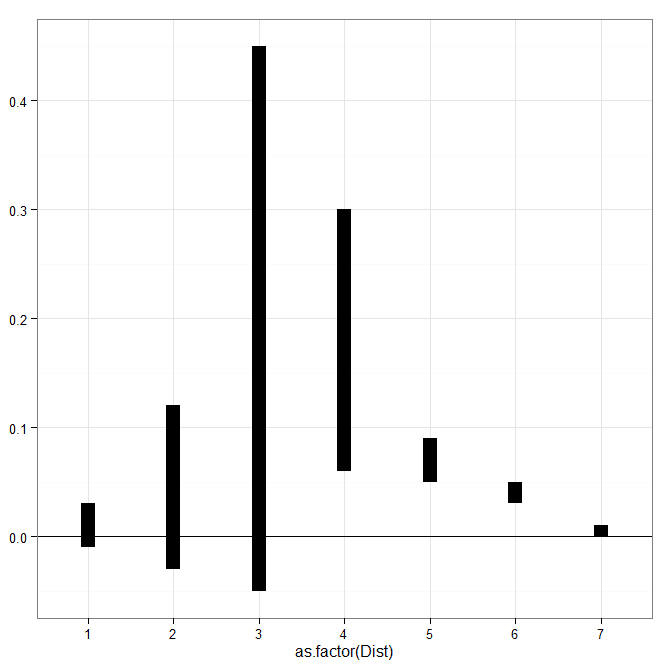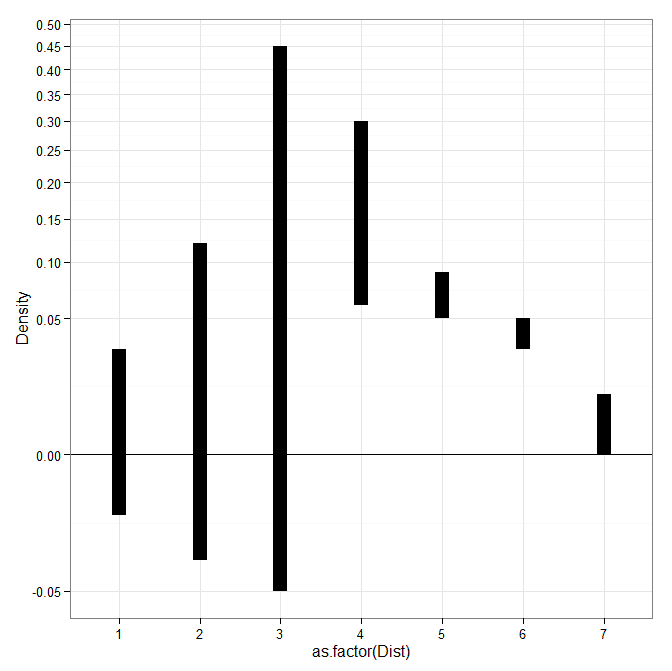# Venn diagrams in R (with some discussion!)

The other day I had a set of three separate categories of binary data that I wanted to visualize with a Venn diagram (or a Euler) diagram of their intersections. I used the `venneuler` R package and it worked out pretty well.

``````library(venneuler)
MyVenn <- venneuler(c(A=74344,B=33197,C=26464,D=148531,"A&B"=11797,
"A&C"=9004,"B&C"=6056,"A&B&C"=2172,"A&D"=0,"A&D"=0,"B&D"=0,"C&D"=0))
MyVenn\$labels <- c("A\n22","B\n7","C\n5","D\n58")
plot(MyVenn)
text(0.59,0.52,"1")
text(0.535,0.51,"3")
text(0.60,0.57,"2")
text(0.64,0.48,"4") ``````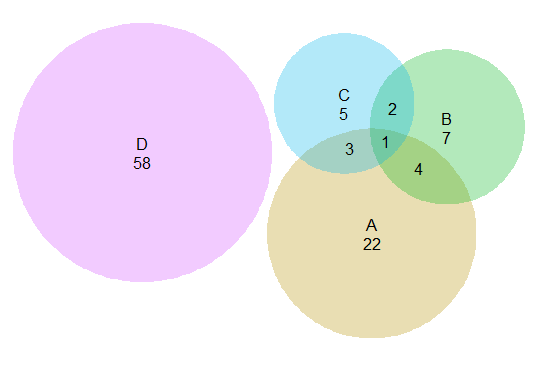Some digging around on this topic though I came across some pretty interesting discussion, in particular a graph makeover of a set of autism diagnoses, see:

for background. Below is a recreated image of the original Venn diagram under discussion (from Kosara’s American Scientist article.)Applying this example to the `venneuler` library did not work out so well.

``````MyVenn2 <- venneuler(c(A=111,B=65,C=94,"A&B"=62,"A&C"=77,"B&C"=52,"A&B&C"=51))
plot(MyVenn2)``````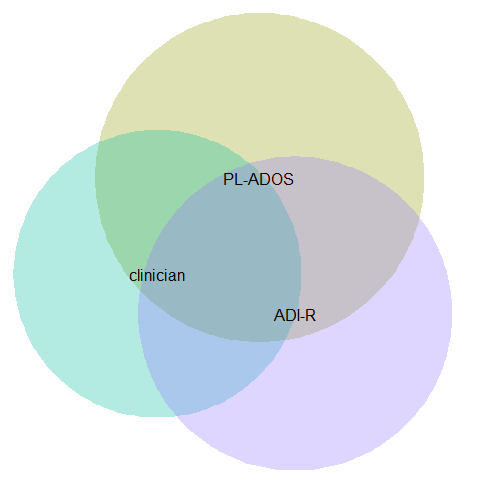Basically there is a limit on the size the intersections can be with the circles, and here the intersection of all three sets is very large, so there is no feasible solution for this example.

This is alittle bit different situation than typical for Venn diagrams though. Typically these charts all one is interested in is the overlaps between each set. But the autism graph that is secondary. What they were really interested in was the sensitivity of the different diagnostic measures (i.e. percentage identifying true positives), and to see if any particular combination had the greatest sensitivity. Although Kosara in his blog post says that all of the redesigns are better than the original I don’t entirely agree, I think Kosara’s version of the Venn diagram with the text labels does a pretty good job, although I think Kosara’s table is sufficient as well. (Kosara’s recreated graph has better labelling than the original Venn diagram, mainly by increasing the relative font size.)

For the autism graph there are basically two over-arching goals:

• identifying the percent within arbitrary multiple intersections
• keeping in mind the baseline N for each of the arbitrary sets

It is not immediately visually obvious, but IMO it is not that hard to arbitrarily collapse different categories in the original Venn diagram and make some rough judgements about the sensitivity. To me the first thing I look at is the center piece, see it is quite a high percentage, and then look to see if I can make any other arbitrary categories to improve upon the sensitivity of all three tests together. All others are either very small baselines or do not improve the percentage, so I conclude that all three combined likely have the most sensitivity. You may also see that the clinicians are quite high for each intersection, so it is questionable whether the two other diagnostics offer any significant improvement over just the clinicians judgement, but many of the clinician sets have quite small N’s, so I don’t put as much stock in that.

Another way to put it is if we think of the original Venn diagram as a graphical table I think it does a pretty good job. The circles and the intersections are a lie factor in the graph, in that their areas do not represent the baseline rates, but it is an intuitive way to lay out the textual categories, and only takes a little work to digest the material. Kosara’s sorted table does a nice job of this as well, but it is easier to ad-hoc combine categories in the Venn diagram than in table rows that are not adjacent. Visually the information does not pop out at you, like a functional relationship in a scatterplot, but the Venn diagram has the ingredients that allow you to drill down and estimate the information you are looking for. Being able to combine arbitrary categories is the key here, and I don’t think any of the other graphical representations allow one to do that very easily.

I thought a useful redesign would be to keep the Venn theme, but have the repeated structures show the base rate via Isotype like recurring graphs. Some of this is motivated by using such diagrams in interpreting statistics (see this post by David Spieghalter for one example, the work of Gerd Gigenzer is relevant as well). I was not able make a nice set of contained glyphs though. Here is a start of what I am talking about, I just exported the R graph into Inkscape and superimposed a bunch of rectangles.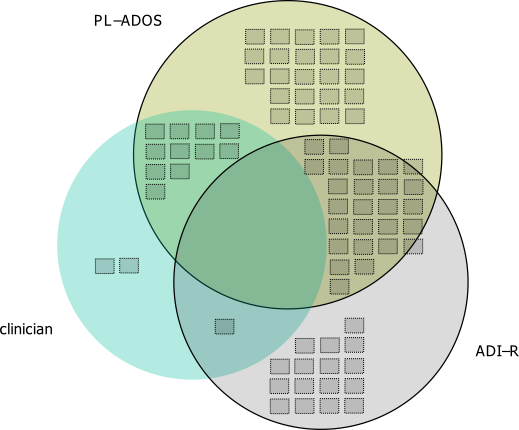This does not visualize the percentage, but one way to do that would be to color or otherwise distinguish the blocks in a certain way. Also I gave up before I finished the intersecting middle piece, and I would need to make the boxes a bit smaller to be able to squeeze it in. I think this idea could be made to work, but this particular example making the Venn even approximately proportional is impossible, and so sticking with the non-proportional Venn diagram and just saying it is not proportional is maybe less likely to be misleading.

I think the idea of using Isotype like repeated structures though could be a generally good idea. Even when the circles can be made to have the areas of the intersection exact, it is still hard to visually gauge the size of circles (rectangles are easier). So the multiple repeated pixels may be more useful anyway, and putting them inside of the circles still allows the arbitrary collapsing of different intersections while still being able to approximately gauge base rates.

# Transforming KDE estimates from Logistic to Probability Scale in R

The other day I had estimates from several logistic regression models, and I wanted to superimpose the univariate KDE’s of the predictions. The outcome was fairly rare, so the predictions were bunched up at the lower end of the probability scale, and the default kernel density estimates on the probability scale smeared too much of the probability outside of the range.

It is a general problem with KDE estimates, and there are two general ways to solve it:

• truncate the KDE and then reweight the points near the edge (example)
• estimate the KDE on some other scale that does not have a restricted domain, and then transform the density back to the domain of interest (example)

The first is basically the same as edge correction in spatial statistics, just in one dimension instead of the two. Here I will show how to do the second in R, mapping items on the logistic scale to the probability scale. The second linked CV post shows how to do this when using the log transformation, and here I will show the same with mapping logistic estimates (e.g. from the output of a logistic regression model). This requires the data to not have any values at 0 or 1 on the probability scale, because these will map to negative and positive infinity on the logistic scale.

In R, first define the logit function as `log(p/(1-p)` and the logistic function as `1/(1+exp(-x))` for use later:

``````logistic <- function(x){1/(1+exp(-x))}
logit <- function(x){log(x/(1-x))}``````

We can generate some fake data that might look like output from a logistic regression model and calculate the density object.

``````set.seed(10)
x <- rnorm(100,0,0.5)
l <- density(x)  #calculate density on logit scale``````

This blog post goes through the necessary math, but in a nut shell you can’t simply just transform the density estimate using the same function, you need to apply an additional transformation (referred to as the Jacobian). So here is an example transforming the density estimate from the logistic scale, `l` above, to the probability scale.

``````px <- logistic(l\$x)  #transform density to probability scale
py <- l\$y/(px*(1-px))
plot(px,py,type='l')``````

To make sure that the area does sum to one, we can superimpose the density calculated on the data transformed to the probability scale. In this example of fake data the two are pretty much identical. (Black line is my transformed density, and the red is the density estimate based on the probability data.)

``````dp <- density(logistic(x)) #density on the probability values to begin with
lines(dp\$x,dp\$y,col='red')``````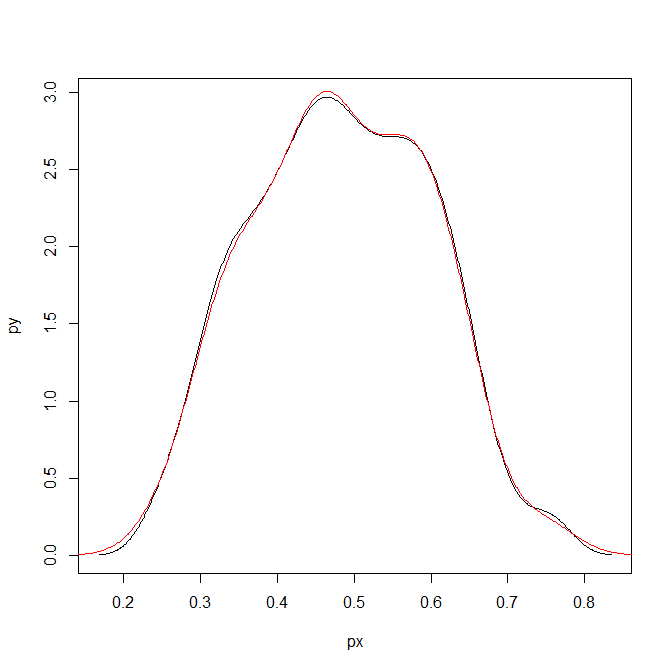Here is a helper function, `denLogistic`, to do this in the future, which simply takes the data (on the logistic scale) and returns a density object modified to the probability scale.

``````logistic <- function(x){1/(1+exp(-x))}
logit <- function(x){log(x/(1-x))}
denLogistic <- function(x){
d <- density(x)
d\$x <- logistic(d\$x)
d\$y <- d\$y/(d\$x*(1-d\$x))
d\$call <- 'Logistic Density Transformed to Probability Scale'
d\$bw <- paste0(signif(d\$bw,4)," (on Logistic scale)")
return(d)
}``````

In cases where more of the probability density is smeared beyond 0-1 on the probability scale, the logistic density estimate will look different. Here is an example with a wider variance and more predictions near zero, so the two estimates differ by a larger amount.

``````lP <- rnorm(100,-0.9,1)
test <- denLogistic(lP)
plot(test)
lines(density(logistic(lP)),col='red')``````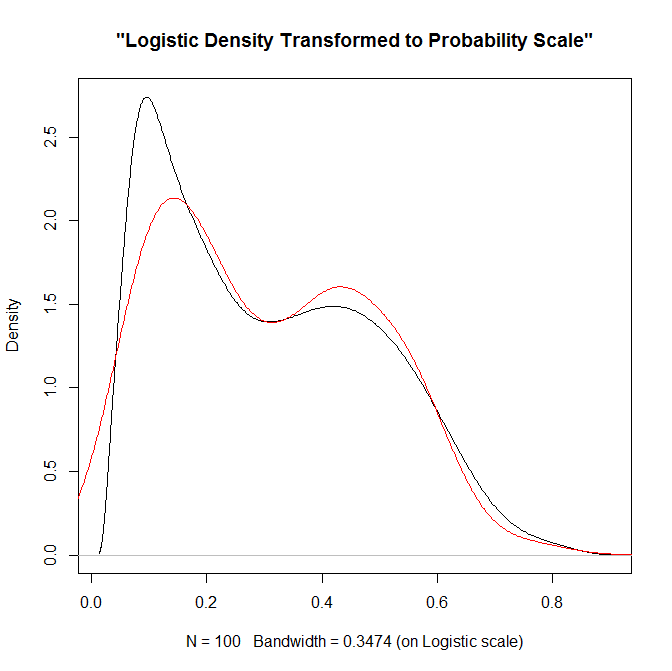Again, this works well for data on the probability scale that can not be exactly zero or one. If you have data like that, the edge correction type KDE estimators are better suited.

# Favorite maps and graphs in historical criminology

I was reading Charles Booth’s Life and Labour of the People in London (available entirely at Google books) and stumbled across this gem of a connected dot plot (between pages 18-19, maybe it came as a fold out in the book?)(You will also get a surprise of the hand of the scanner in the page prior!) This reminded me I wanted to make a collection of my favorite historical examples of maps and graphs for criminology and criminal justice. If you read through Calvin Schmid’s Handbook of Graphical Presentation (available for free at the internet archive) it was a royal pain to create such statistical graphics by hand before computers. It makes you appreciate the effort all that much more, and many of the good ones will rival the quality of any graphic you can make on the computer.

Calvin Schmid himself has some of my favorite example maps. See for instance this gem from Urban Crime Areas: Part II (American Sociological Review, 1960):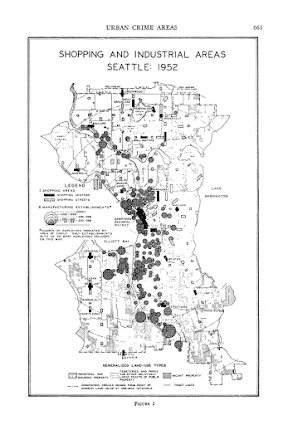The most obvious source of great historical maps in criminology though is from Shaw and McKay’s Juvenile Delinquency in Urban Areas. It was filled with incredible graphs and maps throughout. Here are just a few examples. (These shots are taken from the second edition in 1969, but they are all from the first part of the book, so were likely in the 1942 edition):

Dot maps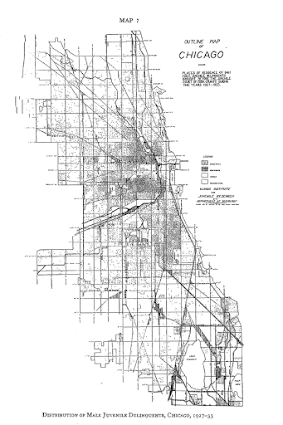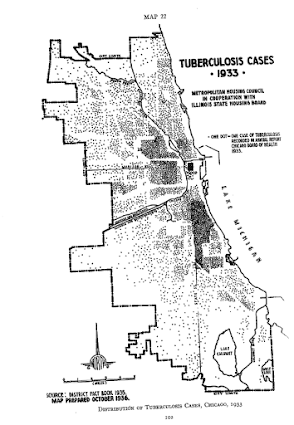Aggregated to grid cells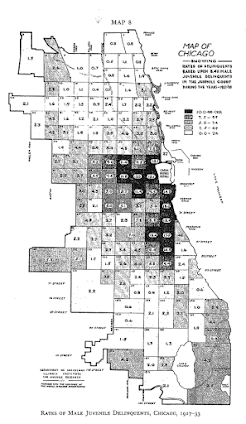The concentric zonal model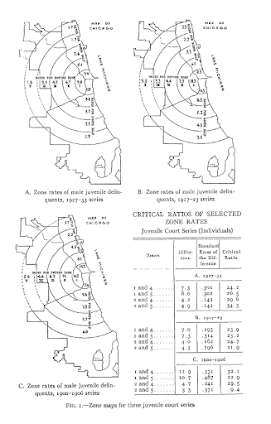And they even have some binned scatterplots to ease in calculating linear regression equations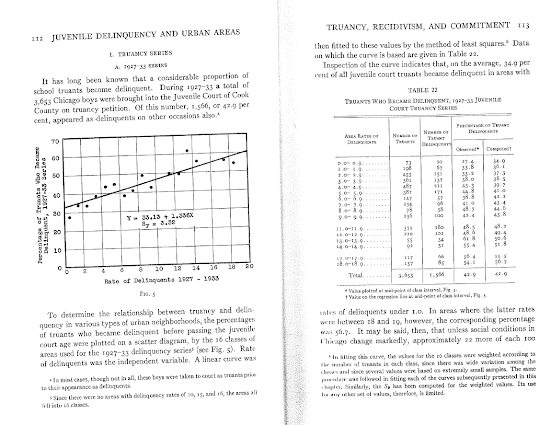Going back further, Friendly in A.-M. Guerry’s moral statistics of France: Challenges for multivariable spatial analysis has some examples of Guerry’s maps and graphs. Besides choropleth maps, Guerry has one of the first examples of a ranked bumps chart (as later coined by Edward Tufte) of the relative rankings of the counts of crime at different ages (1833):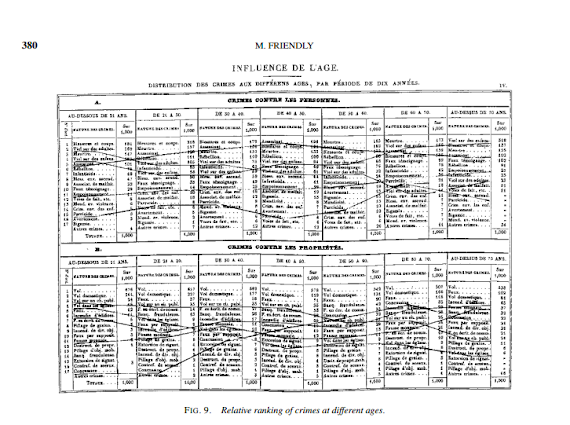I don’t have access to any of Quetelet’s historical maps, but Cook and Wainer in A century and a half of moral statistics in the United Kingdom: Variations on Joseph Fletcher’s thematic maps have examples of Joseph Fletcher’s choropleth maps (as of 1849):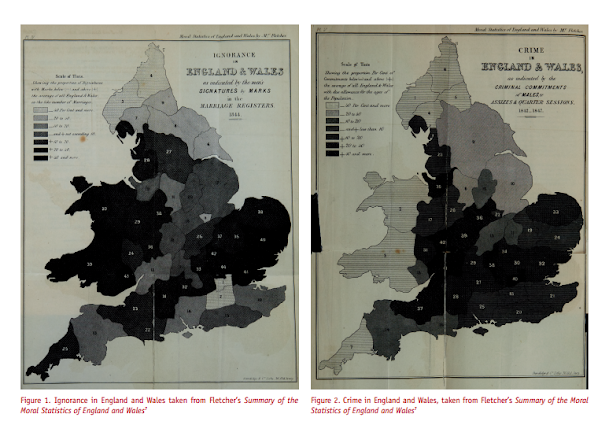Going to more recent mapping examples, the Brantingham’s most notable I suspect is their crime pattern nodes and paths diagram, but my favorites are the ascii glyph contour maps in Crime seen through a cone of resolution (1976):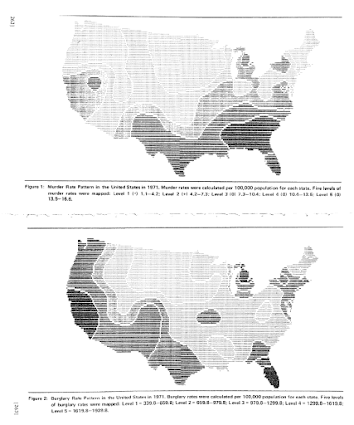The earliest example of a journey-to-crime map I am aware of is Capone and Nichols Urban structure and criminal mobility (1976) (I wouldn’t be surprised though if there are earlier examples)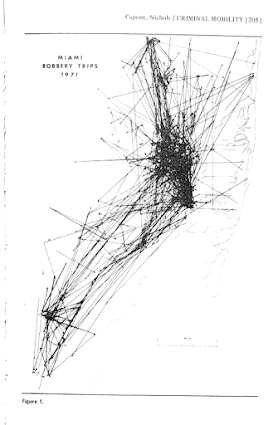Besides maps, one other famous criminology graphic that came to mind was the age-crime curve. This is from Age and the Explanation of Crime (Hirschi and Gottfredson, 1983) (pdf here). This I presume was made with the computer – although I imagine it was still a pain in the butt to do it in 1983 compared to now! Andresen et al.’s reader Classics in Environmental Criminology in the Quetelet chapter has an age crime curve recreated in it (1842), but I will see if I can find an original scan of the image.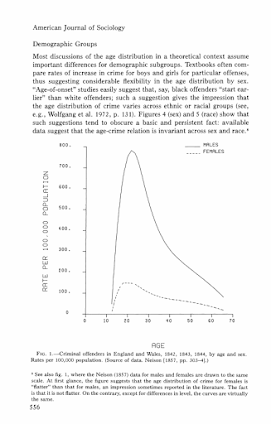I will admit I have not read Wolfgang’s work, but I imagine he had graphs of the empirical cumulative distribution of crime offenses somewhere in Delinquency in a Birth Cohort. But William Spelman has many great examples of them for both people and places. Here is one superimposing the two from Criminal Careers of Public Places (1995):Michael Maltz has spent much work on advocating for visual presentation as well. Here is an example from his chapter, Look Before You Analyze: Visualizing Data in Criminal Justice (pdf here) of a 2.5d kernel density estimate. Maltz discussed this in an earlier publication, Visualizing Homicide: A Research Note (1998), but the image from the book chapter is nicer.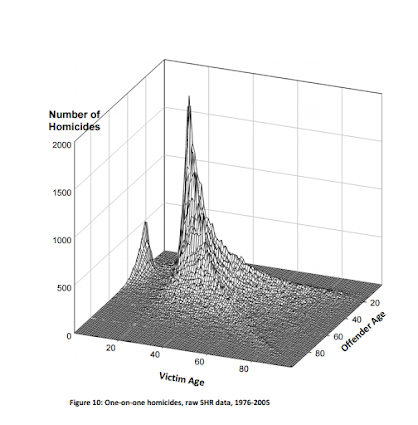Here is an album with all of the images in this post. I will continue to update this post and album with more maps and graphs from historical work in criminology as I find them. I have a few examples in mind — I plan on adding a multivariate scatterplot in Don Newman’s Defensible Space, and I think Sampson’s work in Great American City deserves to be mentioned as well, because he follows in much of the same tradition as Shaw and McKay and presents many simple maps and graphs to illustrate the patterns. I would also like to find the earliest network sociogram of crime relationships. Maltz’s book chapter has a few examples, and Papachristo’s historical work on Al Capone should be mentioned as well (I thought I remembered some nicer network graphs though in Papachristos’s book chapter in the Morselli reader).

Let me know if there are any that I am missing or that you think should be added to the list!

# Some more on Network distances vs Geographic distances intra-city

A prior post on analyzing distances looked at geographic versus network (road) distances between zip codes in New York and one particular location. Over the large distances the correlation ended up being 0.99. But most crime analysis applications will be within one city, so restricting the distances there will the correlation be just as high? I conducted some analysis in Albany, NY to see if this was the case.

First I took a set of 2,640 street segments and intersections in Albany, defined as basically having over 1 reported crime between 2000 through 2013. (This is a pretty good proxy for places where people are actually located in the city, so places where people might actually travel from/to.) Here is a map of those points showing the coverage.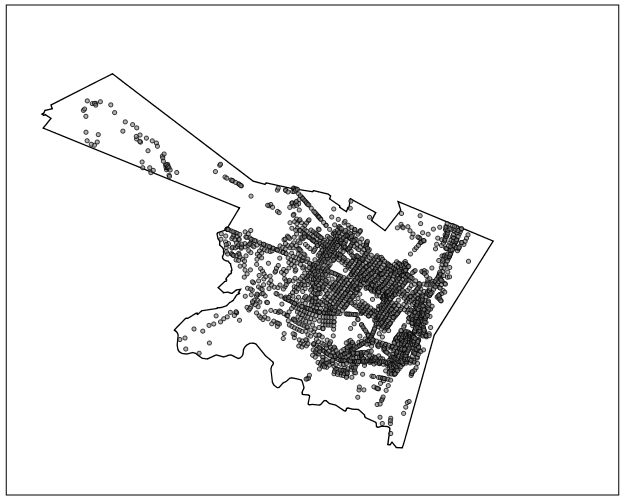I then made the 2,640^2 pairs, and then took a random sample of 2,300 of those pairs to calculate the geographic versus the network distance (calculating the network distance using the google distance API). Here is a flow map, again showing it has pretty good coverage of the city.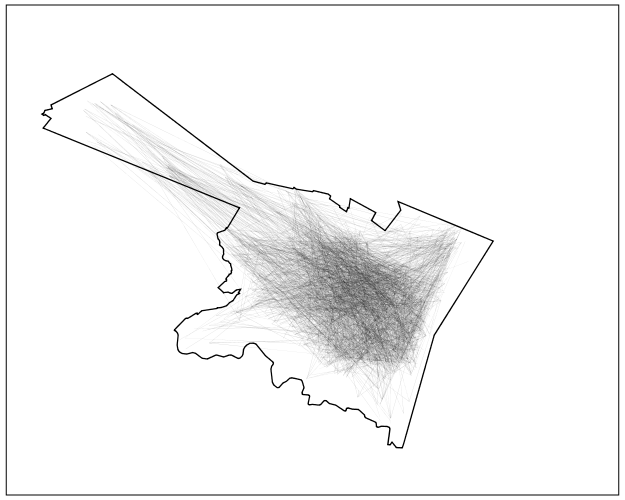In this sample the correlation between the network distance and the geographic distance is 0.94, and below is the scatterplot. The red line is the line of equality, so we can see the network distance is always larger.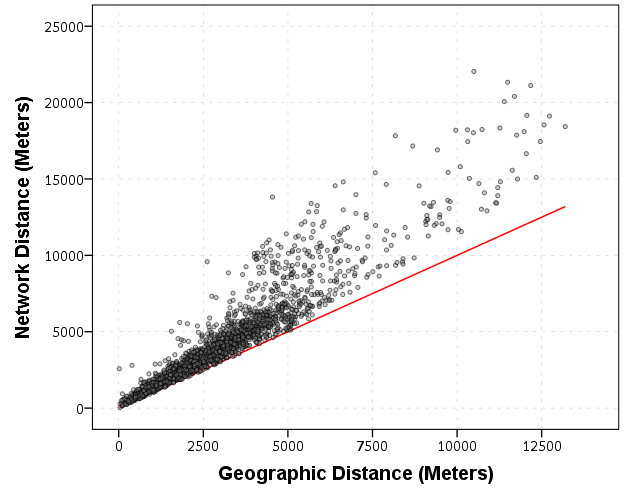Making the graph on log scales basically takes away the heteroscedasticity, and shows some short distance outliers.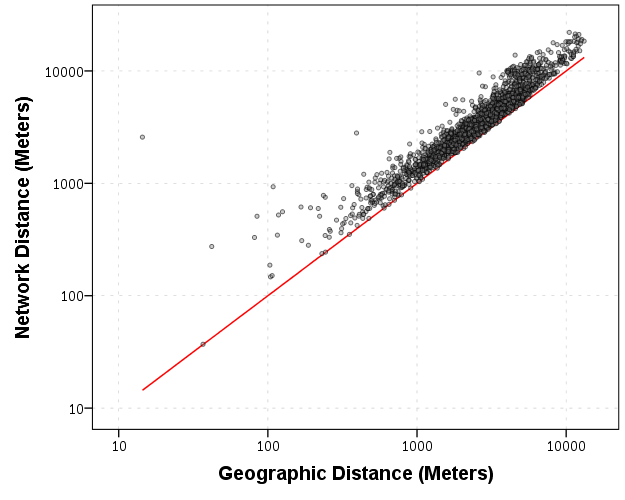I then fit a regression of equation of `log(Network Distance) ~ Intercept + b_0*log(Geo Distance)`, and then calculate the studentized residuals. Here is a small multiple flow map of those locations categorized by the truncated studentized residuals. I plotted flows under 200 meters as a red dot, as otherwise they basically have no area on the map to visualize. There are a few notable patterns, the -1 residuals (so closer network and geo distances) are locations along what looks like Central, Washington, Western and New Scotland (running east-west) and Broadway/Pearl (running north-south). So basically straight, major thoroughfares.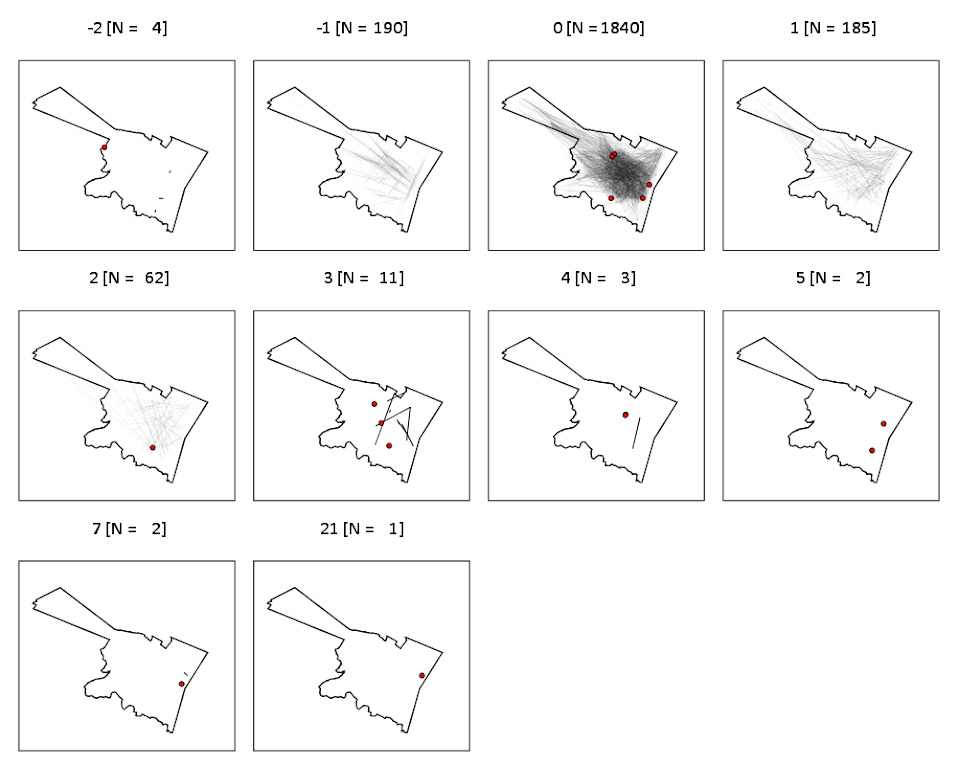It is probable that if more locations in the isthmus and the south western part of the city were selected the distances would be not so nice, but the isthmus itself is largely the Pine Bush park, and the south western part is on the periphery of residential neighborhoods. Exporting the high residuals, what happens in the google distance API is that they are short trips on one way streets, and the to and from and going against the one way. I will have to investigate if you can set the google API to use walking distances to ignore this (as this wasn’t intended as a directed flow like that). Or just learn how to use the CrimeStat or network analysis in ArcMap distance calculation tools!

So although using network distances consistently increases the distances between points, they are still highly correlated, even for shorter in city patterns. If I fixed the flows going against one way streets it would likely be an even higher correlation.